Question

Q21, Q22 and Q23 all refer to the same titration:

Hypochlorous acid has a Ka = 2.9 x10-8. Calculate the pH at the following places on a titration curve when 20.00 mL of 0.200 M HClO is titrated with 0.148 M NaOH.

A) The initial pH.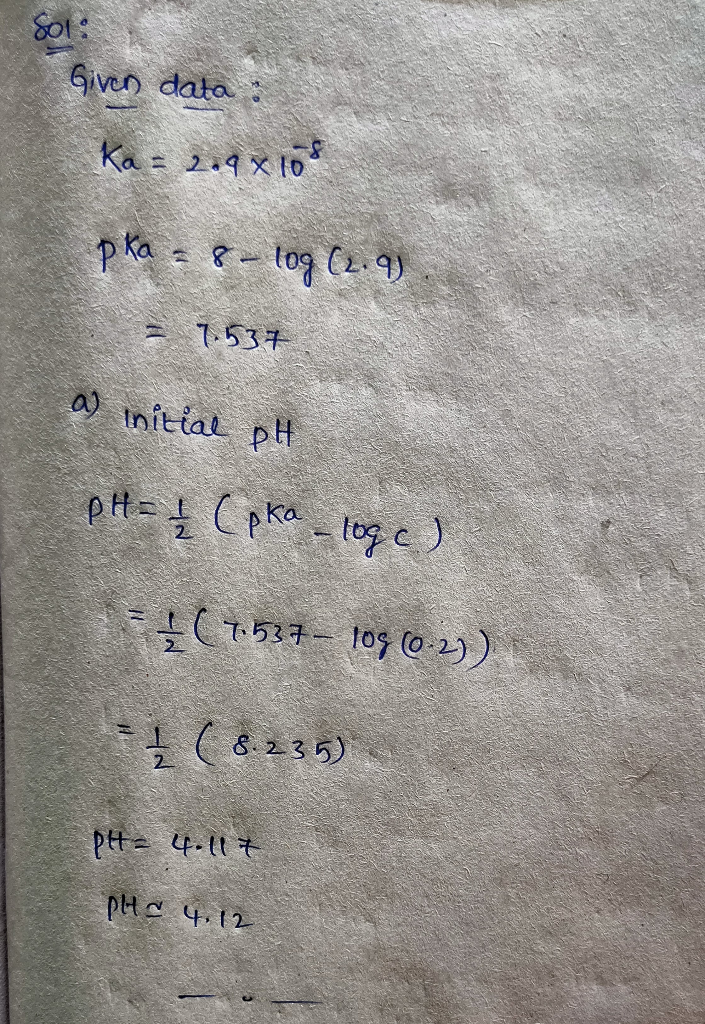#### Earn Coins

Coins can be redeemed for fabulous gifts.

Similar Homework Help Questions
• ### ASAP please Consider the titration of 50.0 mL of 0.200 M hypochlorous acid HCIO (Ka =...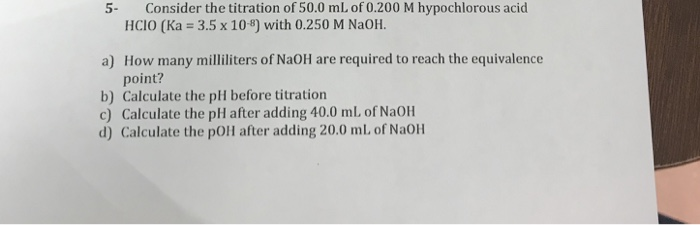ASAP please Consider the titration of 50.0 mL of 0.200 M hypochlorous acid HCIO (Ka = 3.5 x 10-8) with 0.250 M NaOH. 5- How many milliliters of NaOH are required to reach the equivalence point? a) b) Calculate the pH before titration c) Calculate the pH after adding 40.0 mL. of NaOH d) Calculate the pOH after adding 20.0 ml, of NaOH

• ### A 30.0 mL sample of 0.200 M hypochlorous acid (HClO; Ka = 3.0 x 10-8) is...

A 30.0 mL sample of 0.200 M hypochlorous acid (HClO; Ka = 3.0 x 10-8) is titrated with 0.100 M KOH. Calculate the pH after the following volumes have been added 0.0mL 15.0 mL 30.0 mL 45.0 mL 60.0 mL

• ### Of Hydrofluoric acid (Ka-6.6 x 10") solution is titrated with f NaOH. Draw a titration curve, lab...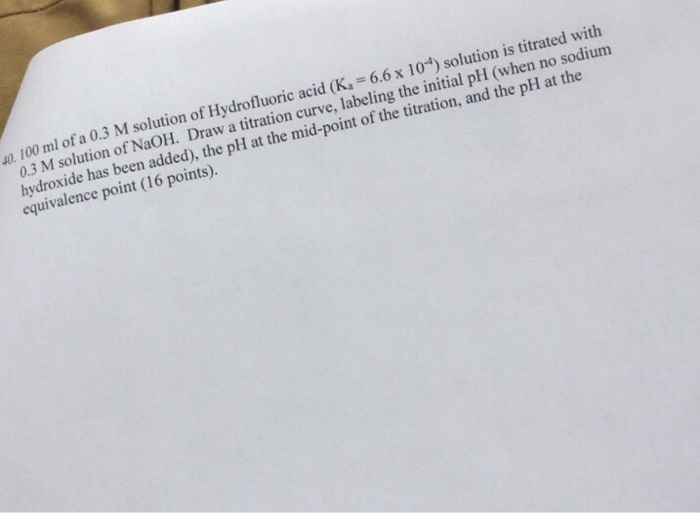of Hydrofluoric acid (Ka-6.6 x 10") solution is titrated with f NaOH. Draw a titration curve, labeling the initial pH (when no sodium 40. 100 ml ofa 0.3 M olution 0.3 M solution hydroxide has been added), the pH at the mid-point of the titration, and the pH at the equivalence point (16 points). of Hydrofluoric acid (Ka-6.6 x 10") solution is titrated with f NaOH. Draw a titration curve, labeling the initial pH (when no sodium 40. 100 ml...

• ### 1)Calculate the pH during the titration of 10.00 mL of 0.400 M hypochlorous acid with 0.500...

1)Calculate the pH during the titration of 10.00 mL of 0.400 M hypochlorous acid with 0.500 M NaOH. First what is the initial pH (before any NaOH is added)? The Ka for HOCl is 3.0 x 10-8 M. 2)How many mL of NaOH are added to reach the equivalence point 3) What is the pH after 2.40 mL of NaOH are added?

• ### Solve a, b and 2 1. Calculate the pH during the titration of 10.00 mL of 0.400 M hypochlorous acid with 0.500 M NaOH. Fi...

Solve a, b and 2 1. Calculate the pH during the titration of 10.00 mL of 0.400 M hypochlorous acid with 0.500 M NaOH. First what is the initial pH (before any NaOH is added)? The Ka for HOCl is 3.0 x 10-8 M. Answer is 3.96 a. What is the pH after 10.60 mL of NaOH are added? b. What is the pH after 15.30 mL of NaOH are added? 2. Chloropropionic acid, ClCH2CH2COOH is a weak monoprotic acid...

• ### IV. Acid-Base Titration (15 points). A 50 mL of 0.200 M HNO2, (Ka= 4.0 x 10)...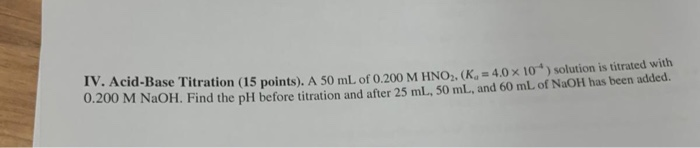IV. Acid-Base Titration (15 points). A 50 mL of 0.200 M HNO2, (Ka= 4.0 x 10) solution is titrated with 0.200 M NaOH. Find the pH before titration and after 25 mL, 50 mL, and 60 mL of NaOH has been added.

• ### Hypochlorous acid has a pKa of 7530. If a 66.0 mL solution of o.180 M is...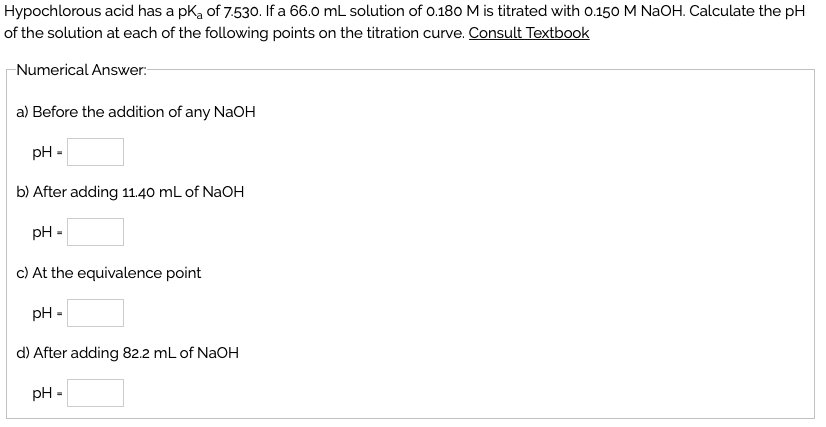Hypochlorous acid has a pKa of 7530. If a 66.0 mL solution of o.180 M is titrated with o.150 M NaOH. Calculate the pH of the solution at each of the following points on the titration curve. Consult Textbook Numerical Answer a) Before the addition of any NaOH pH- b) After adding 11.40 mL of NaOH pH- c) At the equivalence point pH- d) After adding 82.2 mL of NaOH pH-

• ### Calculate the pH during the titration of 10.00 mL of 0.400 M hypochlorous acid with 0.500...

Calculate the pH during the titration of 10.00 mL of 0.400 M hypochlorous acid with 0.500 M NaOH. The Ka for HOCl is 3.0 x 10-8 M. 1. What is the pH after 7.30 mL of NaOH are added? 2. What is the pH after 12.30 mL of NaOH are added? Please use the BCA table.

• ### Hydrofluoric acid has a Ka of 6.8 x 10-4. In this titration, 20.00 mL of 0.120...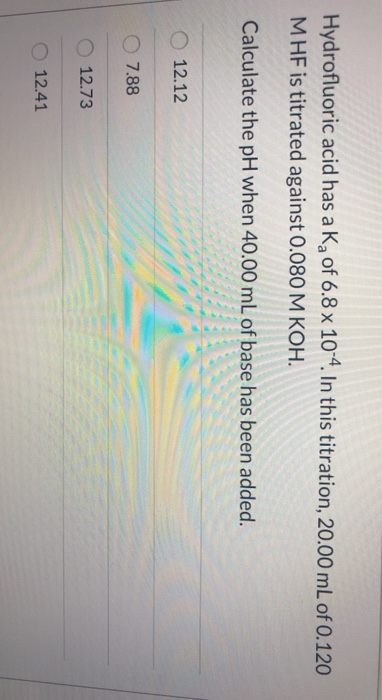Hydrofluoric acid has a Ka of 6.8 x 10-4. In this titration, 20.00 mL of 0.120 MHF is titrated against 0.080 M KOH. Calculate the pH when 40.00 mL of base has been added. 12.12 O 7.88 12.73 12.41

• ### (1) Determine the pH during the titration of 58.4 mL of 0.386 M hypochlorous acid (Ka...

(1) Determine the pH during the titration of 58.4 mL of 0.386 M hypochlorous acid (Ka = 3.5×10-8) by 0.386 M NaOH at the following points. (a) Before the addition of any NaOH (b) After the addition of 15.0 mL of NaOH (c) At the half-equivalence point (the titration midpoint) (d) At the equivalence point (e) After the addition of 87.6 mL of NaOH (2) Determine the pH during the titration of 39.1 mL of 0.369 M ethylamine (C2H5NH2 ,...